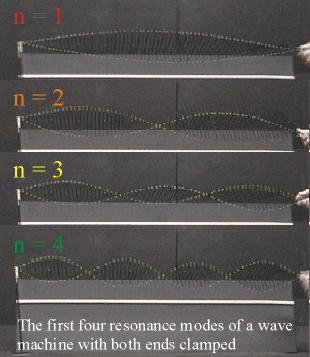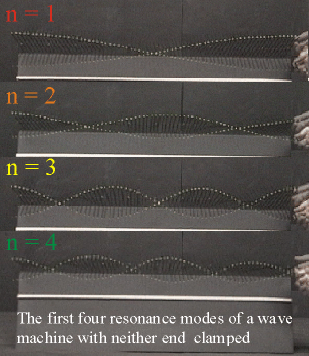### Transverse standing waves with 3 different boundary conditions.

Watch The Video:

Standing waves with two free boundaries

Standing waves with one free boundary

Standing waves with no free boundaries

Teachable Topics:

• oscillations& frequency
• resonance
• standing waves and nodes
• sound and music

Theory:

A wave sent down along the wave machine towards a clamped end will reflect off that end and travel back toward its origin. If other waves have already been created, then the first wave will interfere with these as it travels into them. At certain frequencies, this interference produces standing waves - wave patterns that don't travel along the length of the wave machine. Such a pattern has points called nodes where the medium is always at rest and anti-nodes where the wave amplitude is a maximum.

There can be three different cases. In the first case, both ends of the wave machine are held in place, so there is a node at each end. Therefore, the only standing waves that can exist are ones where the wave machine's length (L) is an whole number (n) of half-wavelengths (where one wavelength has the symbol λ). In symbolic form, this means

L = n(λ/2)

Both ends of the wave machine need not be clamped for standing waves to be created. One end can be oscillated by hand (if the oscillations are small, it is almost as if there is a node at this end), while the other end can be free (so it becomes an anti-node). This is the second case, and standing waves must now be such that the length of the wave machine is an odd multiple of a quarter wavelength.

L = (2n + 1)(λ/4)

NOTE: Though n = 0 is a possible resonance mode, it is difficult to produce on the wave machine, and thus doesn't appear in the video. Also, because n = 0 is the lowest mode here, it is referred to as the "first resonance mode", n = 1 is referred to as the "second resonance mode", and so on.

L = n(λ/2)

The last kind of standing wave pattern occurs when each end is free (thus both are anti-nodes). Now the standing waves are such that the length of the wave machine is a whole number of half-wavelengthsFigure 1: Resonant frequencies with clamped endsFigure 2: Resonant frequencies with unclamped ends

Apparatus:

• The small wave machine
• The terminal clamp

Procedure:

Two clamps:

For the first part, place a terminal clamp at the end of the small wave machine and hold the other end. Even though your hand will be oscillating the rods, the rod you hold will be a node if the oscillations are kept small. By trial and error you can create the first few (four or five) resonance modes. Some practice is needed to get a “feel” for the various frequencies.

##### One clamp:

Remove the clamp and again use your hand to oscillate the wave machine. Again, the rod you hold should be forced to be a node. Since the other end is unconstrained, it acts as an anti-node in the four or five resonance modes that can be established.

##### No clamps:

Another way to produce a standing wave is by having anti-nodes at both ends. Loosely hold the last rod you were holding before, and oscillate the wave machine. Doing this is much more challenging than making standing waves in any of the previous setups, and more practice is required.

Note: There are two wave machines - a wide one and a thinner one. This thinner one is the "small wave machine" and is a lot easier to use for this demo.